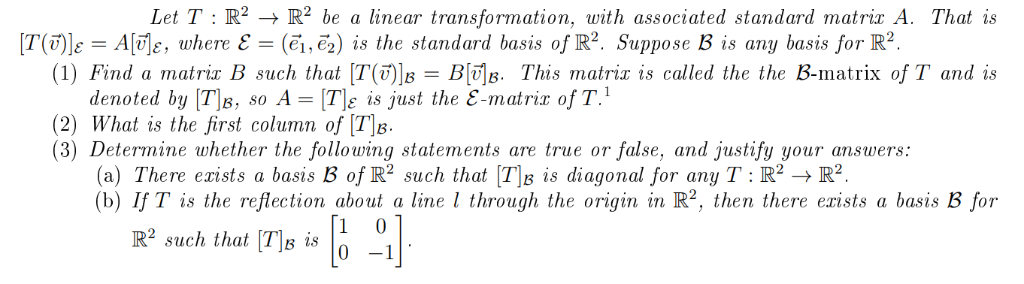# Solved: Let T R2 - R2 Be A Linear Transformation, With Associated Standard Matric A. That Is (?1,e2) Is The Standard Basis Of R2. Suppose B Is Any Basis For R2. T(5)]e = A[vje, Where E = (1) Find A Ma

By |Let T R2 - R2 be a linear transformation, with associated standard matric A. That is (?1,e2) is the standard basis of R2. Suppose B is any basis for R2. T(5)]e = A[vje, where E = (1) Find a matrix B such that [T()B = BB. This matrix is called the the B-matrix of T and is denoted by T8, so A = [T\e is just the E-matrix of T. (2) What is the first column of [T\B. (3) Determine whether the following statements are true or false, and justify your answers (a) There exists a basis B of R2 such that [T\B (b) If T is the reflection about a line l through the origin in R2, then there exists a basis B for diagonal for any T : R2 ->R2. R2 such that TB is